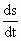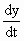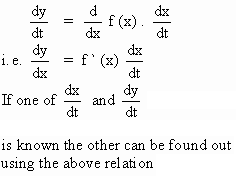Home MonkeyNotes Printable Notes Digital Library Study Guides Study Smart Parents Tips College Planning Test Prep Fun Zone Help / FAQ How to Cite New Title Request

5.14 Related Rates

We have seen thatis the derivative of s (t) w. r. to t and can be interpreted as the velocity.

This physical interpretation can be extended further.

If (x, y) are the co-ordinates of a moving particle then both x and y are functions of  t ., then, is the rate of change of x w. r. to  t  andis the rate of change of y w. r. to  tsince both x and y are related by a function y = f (x),are also related. If one of is known the other can be found using the relation between these rates.

Problem of this type are known as problem of related rates.Your browser does not support the IFRAME tag.

Technique : y = f (x) where both x and y are dependent on time ' t '

HenceIndex

5.1 Tangent And Normal Lines
5.2 Angle Between Two Curves
5.3 Interpretation Of The Sign Of The Derivative
5.4 Locality Increasing Or Decreasing Functions 5.5 Critical Points
5.6 Turning Points
5.7 Extreme Value Theorem
5.8 The Mean-value Theorem
5.9 First Derivative Test For Local Extrema
5.10 Second Derivative Test For Local Extrema
5.11 Stationary Points
5.12 Concavity And Points Of Inflection
5.13 Rate Measure (distance, Velocity And Acceleration)
5.14 Related Rates
5.15 Differentials : Errors And Approximation